# Geometry

## サマリー

Geometry objects define a spatial location and an associated geometric shape.

## 構文

` Geometry  (geometry, inputs, {spatial_reference}, {has_z}, {has_m})`
 パラメーター 説明 データ タイプ geometry The geometry type: point, polygon, polyline, or multipoint. String inputs The coordinates used to create the object. The data type can be either Point or Array objects. Object spatial_reference The spatial reference of the new geometry.(デフォルト値は次のとおりです None) SpatialReference has_z The Z state: True for geometry if Z is enabled and False if it is not. (デフォルト値は次のとおりです False) Boolean has_m The M state: True for geometry if M is enabled and False if it is not.(デフォルト値は次のとおりです False) Boolean

## プロパティ

プロパティ説明データ タイプ
JSON
(読み取り専用)

Returns an Esri JSON representation of the geometry as a string.

##### ヒント:

The returned string can be converted to a dictionary using the json module's loads function.

String
WKB
(読み取り専用)

Returns the well-known binary (WKB) representation for OGC geometry. It provides a portable representation of a geometry value as a contiguous stream of bytes.

Bytearray
WKT
(読み取り専用)

Returns the well-known text (WKT) representation for OGC geometry. It provides a portable representation of a geometry value as a text string.

Any true curves in the geometry will be densified into approximate curves in the WKT string.

String
area
(読み取り専用)

The area of a polygon feature. It is zero for all other feature types.

Double
centroid
(読み取り専用)

The true centroid if it is within or on the feature; otherwise, the label point is returned.

Point
extent
(読み書き)

The extent of the geometry.

Extent
firstPoint
(読み取り専用)

The first coordinate point of the geometry.

Point
hasCurves
(読み取り専用)

Returns True if the geometry has a curve.

Boolean
hullRectangle
(読み取り専用)

A space-delimited string of the coordinate pairs of the convex hull rectangle.

String
isMultipart
(読み取り専用)

Returns True if the number of parts for this geometry is more than one.

Boolean
labelPoint
(読み取り専用)

The point at which the label is located. The labelPoint is always located within or on a feature.

Point
lastPoint
(読み取り専用)

The last coordinate of the feature.

Point
length
(読み取り専用)

The length of the linear feature. It is zero for point and multipoint feature types.

Double
length3D
(読み書き)

The 3D length of the linear feature. It is zero for point and multipoint feature types.

Double
partCount
(読み取り専用)

The number of geometry parts for the feature.

Integer
pointCount
(読み取り専用)

The total number of points for the feature.

Integer
spatialReference
(読み取り専用)

The spatial reference of the geometry.

SpatialReference
trueCentroid
(読み取り専用)

The center of gravity for a feature.

Point
type
(読み取り専用)

The geometry type: polygon, polyline, point, multipoint, multipatch, dimension, or annotation.

String

## 方法の概要

 方法 説明 angleAndDistanceTo (other, {method}) Returns a tuple of angle and distance to another point using a measurement type. boundary () Constructs the boundary of the geometry.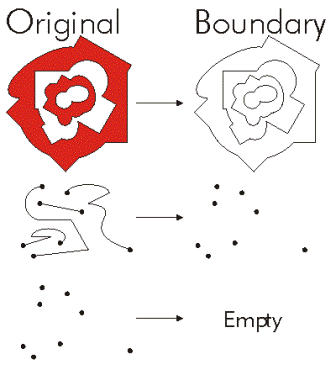buffer (distance) Constructs a polygon at a specified distance from the geometry.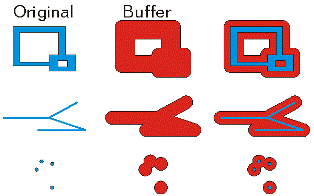clip (envelope) Constructs the intersection of the geometry and the specified extent.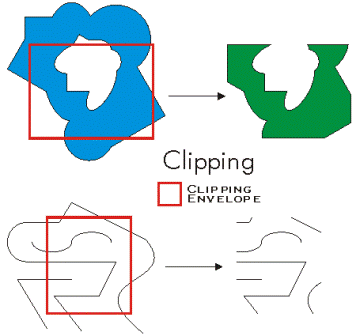contains (second_geometry, {relation}) Indicates if the base geometry contains the comparison geometry. contains is the opposite of within. Only True relationships are shown in this illustration.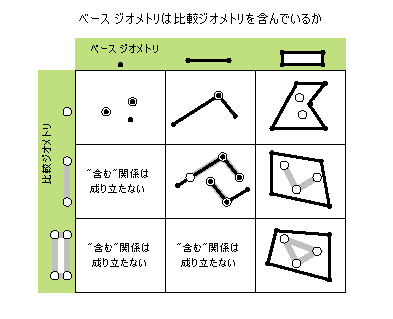convexHull () Constructs the geometry that is the minimal bounding polygon such that all outer angles are convex.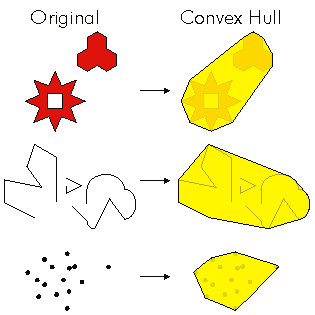crosses (second_geometry) Indicates if the two geometries intersect in a geometry of a lesser shape type. Two polylines cross if they share only points in common, at least one of which is not an endpoint. A polyline and an polygon cross if they share a polyline or a point (for vertical line) in common on the interior of the polygon which is not equivalent to the entire polyline. Only True relationships are shown in this illustration.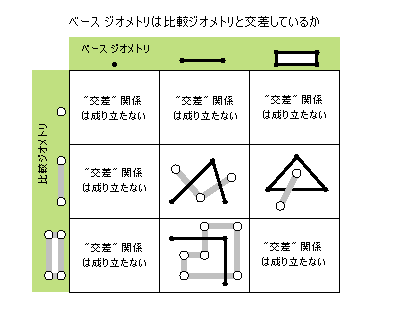cut (cutter) Splits this geometry into a part left of the cutting polyline, and a part right of it. When a polyline or polygon is cut, it is split where it intersects the cutter polyline. Each piece is classified as left of or right of the cutter. This classification is based on the orientation of the cutter line. Parts of the target polyline that do not intersect the cutting polyline are returned as part of the right of result for that input polyline. If a geometry is not cut, the left geometry will be empty (None).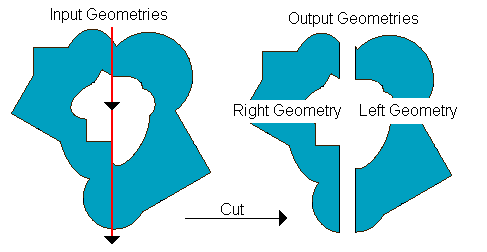densify (type, distance, {deviation}) Creates a new geometry with added vertices. difference (other) Constructs the geometry that is composed only of the region unique to the base geometry but not part of the other geometry. The following illustration shows the results when the red polygon is the source geometry.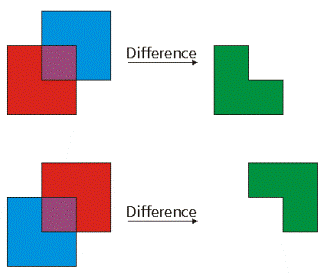disjoint (second_geometry) Indicates if the base and comparison geometries share no points in common. Two geometries intersect if disjoint returns False. Only True relationships are shown in this illustration.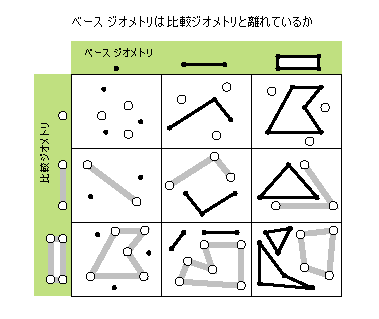distanceTo (other) Returns the minimum distance between two geometries. The distance is in the units of the geometry's spatial reference. If the geometries intersect, the minimum distance is 0.Both geometries must have the same projection. equals (second_geometry) Indicates if the base and comparison geometries are of the same shape type and define the same set of points in the plane. This is a 2D comparison only; M and Z values are ignored.Only True relationships are shown in this illustration.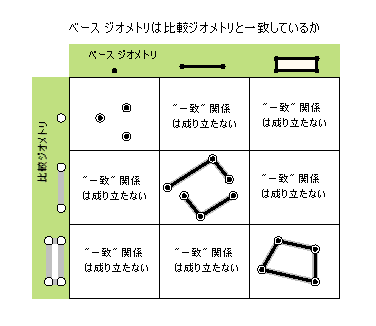generalize (max_offset) Creates a new simplified geometry using a specified maximum offset tolerance. getArea ({type}, {units}) Returns the area of the feature using a measurement type. getLength ({measurement_type}, {units}) Returns the length of the feature using a measurement type. getPart ({index}) Returns an Array object of Point objects for a particular part of the geometry if an index is specified. If an index is not specified, an Array object containing an Array of Point objects for each geometry part is returned. The getPart method is equivalent to indexing an object; that is, obj.getPart(0) is equivalent to obj. intersect (other, dimension) Constructs a geometry that is the geometric intersection of the two input geometries. Different dimension values can be used to create different shape types. The intersection of two geometries of the same shape type is a geometry containing only the regions of overlap between the original geometries.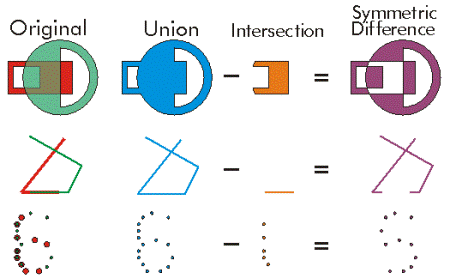For faster results, test if the two geometries are disjoint before calling intersect. measureOnLine (in_point, {use_percentage}) Returns a measure from the start point of this line to the in_point. overlaps (second_geometry) Indicates if the intersection of the two geometries has the same shape type as one of the input geometries and is not equivalent to either of the input geometries. Only True relationships are shown in this illustration.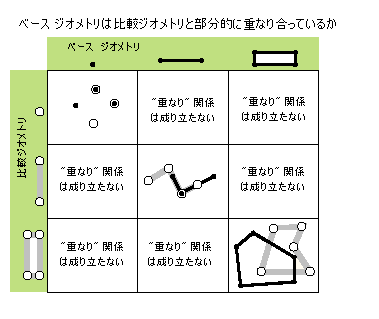pointFromAngleAndDistance (angle, distance, {method}) Returns a point at a given angle in degrees and distance in the units of the geometry's spatial reference using the specified measurement type. positionAlongLine (value, {use_percentage}) Returns a point on a line at a specified distance from the beginning of the line. projectAs (spatial_reference, {transformation_name}) Projects a geometry and optionally applies a geotransformation. To project, the geometry must have a spatial reference, and not have an unknown coordinate system. The new spatial reference system passed to the method defines the output coordinate system. If either spatial reference is unknown, the coordinates will not be changed. The z- and m-values are not changed by the projectAs method. queryPointAndDistance (in_point, {as_percentage}) Finds the point on the polyline nearest to the in_point and the distance between those points. Also returns information about the side of the line the in_point is on as well as the distance along the line where the nearest point occurs. segmentAlongLine (start_measure, end_measure, {use_percentage}) Returns a Polyline between start and end measures. Similar to Polyline.positionAlongLine but will return a polyline segment between two points on the polyline instead of a single point. snapToLine (in_point) Returns a new point based on in_point snapped to this geometry. symmetricDifference (other) Constructs the geometry that is the union of two geometries minus the instersection of those geometries.The two input geometries must be the same shape type.touches (second_geometry) Indicates if the boundaries of the geometries intersect. Two geometries touch when the intersection of the geometries is not empty, but the intersection of their interiors is empty. For example, a point touches a polyline only if the point is coincident with one of the polyline end points. Only True relationships are shown in this illustration.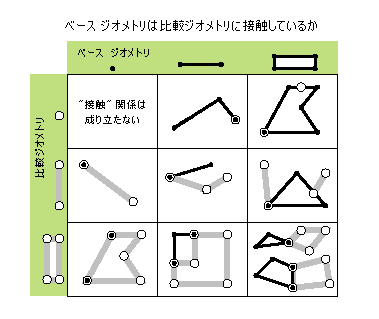union (other) Constructs the geometry that is the set-theoretic union of the input geometries. The two geometries being unioned must be the same shape type.within (second_geometry, {relation}) Indicates if the base geometry is within the comparison geometry. within is the opposite operator of contains. Only True relationships are shown in this illustration.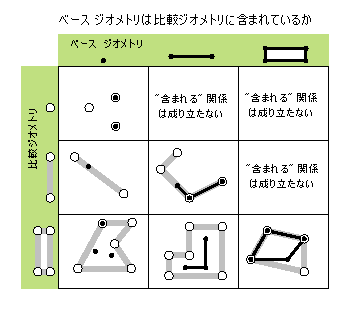The base geometry is within the comparison geometry if the base geometry is the intersection of the geometries and the intersection of their interiors is not empty. within is a Clementini operator, except in the case of an empty base geometry.

## 方法

`angleAndDistanceTo (other, {method})`
 パラメーター 説明 データ タイプ other The second geometry. PointGeometry method PLANAR measurements reflect the projection of geographic data onto the 2D surface (in other words, they will not take into account the curvature of the earth). GEODESIC, GREAT_ELLIPTIC, LOXODROME, and PRESERVE_SHAPE measurement types may be chosen as an alternative, if desired. GEODESIC —The shortest line between any two points on the earth's surface on a spheroid (ellipsoid). One use for a geodesic line is when you want to determine the shortest distance between two cities for an airplane's flight path. This is also known as a great circle line if based on a sphere rather than an ellipsoid.GREAT_ELLIPTIC —The line on a spheroid (ellipsoid) defined by the intersection at the surface by a plane that passes through the center of the spheroid and the start and endpoints of a segment. This is also known as a great circle when a sphere is used.LOXODROME —A loxodrome is not the shortest distance between two points but instead defines the line of constant bearing, or azimuth. Great circle routes are often broken into a series of loxodromes, which simplifies navigation. This is also known as a rhumb line.PLANAR —Planar measurements use 2D Cartesian mathematics to calculate lengths and areas. This option is only available when measuring in a projected coordinate system and the 2D plane of that coordinate system will be used as the basis for the measurements.PRESERVE_SHAPE —This type calculates the area or length of the geometry on the surface of the earth ellipsoid, for geometry defined in a projected or geographic coordinate system. This option preserves the shape of the geometry in its coordinate system.(デフォルト値は次のとおりです GEODESIC) String

 データ タイプ 説明 tuple Returns a tuple of angle (in degrees) and distance (in meters) to another point.
`boundary ()`

 データ タイプ 説明 Object A polygon's boundary is a polyline. A polyline's boundary is a multipoint, corresponding to the endpoints of the line. A point or multipoint's boundary is an empty point or multipoint.
`buffer (distance)`
 パラメーター 説明 データ タイプ distance The buffer distance.The buffer distance is in the same units as the geometry that is being buffered. A negative distance can only be specified against a polygon geometry. Double

 データ タイプ 説明 Polygon The buffered polygon geometry.
`clip (envelope)`
 パラメーター 説明 データ タイプ envelope An Extent object used to define the clip extent. Extent

 データ タイプ 説明 Object An output geometry clipped to the specified extent.
`contains (second_geometry, {relation})`
 パラメーター 説明 データ タイプ second_geometry A second geometry. Object relation The spatial relationship type.BOUNDARY — Relationship has no restrictions for interiors or boundaries.CLEMENTINI — Interiors of geometries must intersect. Specifying CLEMENTINI is equivalent to specifying None. This is the default.PROPER — Boundaries of geometries must not intersect.(デフォルト値は次のとおりです None) String

 データ タイプ 説明 Boolean A return Boolean value of True indicates this geometry contains the second geometry.
`convexHull ()`

 データ タイプ 説明 Object The resulting geometry. The convex hull of a single point is the point itself.
`crosses (second_geometry)`
 パラメーター 説明 データ タイプ second_geometry A second geometry. Object

 データ タイプ 説明 Boolean A return Boolean value of True indicates the two geometries intersect in a geometry of a lesser shape type.
`cut (cutter)`
 パラメーター 説明 データ タイプ cutter The cutting polyline geometry. PolyLine

 データ タイプ 説明 Geometry A list of two geometries.
`densify (type, distance, {deviation})`
 パラメーター 説明 データ タイプ type The type of densification, DISTANCE, ANGLE, or GEODESIC. DISTANCE —Creates a new feature that is a piecewise linear approximation of the input. ANGLE —Creates a new feature that is a piecewise linear approximation of the input. Vertices are introduced at points where the angle between tangents at those points is the provided angle. GEODESIC —Densifies and reshapes segments between input vertices so that the output segments follow the shortest ground path connecting input vertices. String distance The maximum distance between vertices. The actual distance between vertices will usually be less than the maximum distance as new vertices will be evenly distributed along the original segment. If using a type of DISTANCE or ANGLE, the distance is measured in the units of the geometry's spatial reference. If using a type of GEODESIC, the distance is measured in meters. Double deviation Densify uses straight lines to approximate curves. You use deviation to control the accuracy of this approximation. The deviation is the maximum distance between the new segment and the original curve. The smaller its value, the more segments will be required to approximate the curve. If using a type of DISTANCE, the deviation is measured in the units of the geometry's spatial reference. If using a type of ANGLE, the deviation is measured in radians. If using a type of GEODESIC, the deviation is not used. Double

 データ タイプ 説明 Geometry The densified geometry.
`difference (other)`
 パラメーター 説明 データ タイプ other A second geometry. Object

 データ タイプ 説明 Object The resulting geometry.
`disjoint (second_geometry)`
 パラメーター 説明 データ タイプ second_geometry A second geometry. Object

 データ タイプ 説明 Boolean A return Boolean value of True indicates that the two geometries share no points in common.
`distanceTo (other)`
 パラメーター 説明 データ タイプ other A second geometry. Object

 データ タイプ 説明 Double The distance between the two geometries.
`equals (second_geometry)`
 パラメーター 説明 データ タイプ second_geometry A second geometry. Object

 データ タイプ 説明 Boolean A return Boolean value of True indicates that the two geometries are of the same shape type and define the same set of points in the plane.
`generalize (max_offset)`
 パラメーター 説明 データ タイプ max_offset The maximum offset tolerance. Double

 データ タイプ 説明 Geometry The generalized geometry.
`getArea ({type}, {units})`
 パラメーター 説明 データ タイプ type PLANAR measurements reflect the projection of geographic data onto the 2D surface (in other words, they will not take into account the curvature of the earth). GEODESIC, GREAT_ELLIPTIC, LOXODROME, and PRESERVE_SHAPE measurement types may be chosen as an alternative, if desired. GEODESIC —The shortest line between any two points on the earth's surface on a spheroid (ellipsoid). One use for a geodesic line is when you want to determine the shortest distance between two cities for an airplane's flight path. This is also known as a great circle line if based on a sphere rather than an ellipsoid.GREAT_ELLIPTIC —The line on a spheroid (ellipsoid) defined by the intersection at the surface by a plane that passes through the center of the spheroid and the start and endpoints of a segment. This is also known as a great circle when a sphere is used.LOXODROME —A loxodrome is not the shortest distance between two points but instead defines the line of constant bearing, or azimuth. Great circle routes are often broken into a series of loxodromes, which simplifies navigation. This is also known as a rhumb line.PLANAR —Planar measurements use 2D Cartesian mathematics to calculate lengths and areas. This option is only available when measuring in a projected coordinate system and the 2D plane of that coordinate system will be used as the basis for the measurements.PRESERVE_SHAPE —This type calculates the area or length of the geometry on the surface of the earth ellipsoid, for geometry defined in a projected or geographic coordinate system. This option preserves the shape of the geometry in its coordinate system.(デフォルト値は次のとおりです GEODESIC) String units The units in which the area will be calculated.ACRES —AcresARES —AresHECTARES —HectaresSQUARECENTIMETERS —Square centimetersSQUAREDECIMETERS —Square decimetersSQUAREFEET —Square feetSQUAREINCHES —Square inchesSQUAREKILOMETERS —Square kilometersSQUAREMETERS —Square metersSQUAREMILES —Square milesSQUAREMILLIMETERS —Square millimetersSQUAREYARDS —Square yards String

 データ タイプ 説明 Double The area of the feature.By default, for Projected coordinate systems, area will be returned in the units of the coordinate system, and for Geographic coordinate systems, area will be returned in square meters.
`getLength ({measurement_type}, {units})`
 パラメーター 説明 データ タイプ measurement_type PLANAR measurements reflect the projection of geographic data onto the 2D surface (in other words, they will not take into account the curvature of the earth). GEODESIC, GREAT_ELLIPTIC, LOXODROME, and PRESERVE_SHAPE measurement types may be chosen as an alternative, if desired.GEODESIC —The shortest line between any two points on the earth's surface on a spheroid (ellipsoid). One use for a geodesic line is when you want to determine the shortest distance between two cities for an airplane's flight path. This is also known as a great circle line if based on a sphere rather than an ellipsoid.GREAT_ELLIPTIC —The line on a spheroid (ellipsoid) defined by the intersection at the surface by a plane that passes through the center of the spheroid and the start and endpoints of a segment. This is also known as a great circle when a sphere is used.LOXODROME —A loxodrome is not the shortest distance between two points but instead defines the line of constant bearing, or azimuth. Great circle routes are often broken into a series of loxodromes, which simplifies navigation. This is also known as a rhumb line.PLANAR —Planar measurements use 2D Cartesian mathematics to calculate lengths and areas. This option is only available when measuring in a projected coordinate system and the 2D plane of that coordinate system will be used as the basis for the measurements.PRESERVE_SHAPE —This type calculates the area or length of the geometry on the surface of the earth ellipsoid, for geometry defined in a projected or geographic coordinate system. This option preserves the shape of the geometry in its coordinate system.(デフォルト値は次のとおりです GEODESIC) String units The units in which the length will be calculated.CENTIMETERS —CentimetersDECIMETERS —DecimetersFEET —FeetINCHES —InchesKILOMETERS —KilometersMETERS —MetersMILES —MilesMILLIMETERS —MillimetersNAUTICALMILES —Nautical MilesYARDS —Yards String

 データ タイプ 説明 Double The length of the feature.By default, for Projected coordinate systems, length will be returned in the units of the coordinate system, and for Geographic coordinate systems, length will be returned in square meters.
`getPart ({index})`
 パラメーター 説明 データ タイプ index The index position of the geometry. Integer

 データ タイプ 説明 Array The resultant Array object.
`intersect (other, dimension)`
 パラメーター 説明 データ タイプ other The second geometry. Object dimension The topological dimension (shape type) of the resulting geometry. 1 —A zero-dimensional geometry (point or multipoint). 2 —A one-dimensional geometry (polyline). 4 —A two-dimensional geometry (polygon). Integer

 データ タイプ 説明 Object A new geometry (point, multipoint, polyline, or polygon) that is the geometric intersection of the two input geometries.
`measureOnLine (in_point, {use_percentage})`
 パラメーター 説明 データ タイプ in_point A point (PointGeometry or Point) that is used to measure from the start point of the polyline. PointGeometry use_percentage If False, the measure will be returned as a distance; if True, the measure will be returned as a percentage.(デフォルト値は次のとおりです False) Boolean

 データ タイプ 説明 Double A distance or percentage.
`overlaps (second_geometry)`
 パラメーター 説明 データ タイプ second_geometry A second geometry. Object

 データ タイプ 説明 Boolean A return Boolean value of True indicates the intersection of the two geometries has the same dimension as one of the input geometries.
`pointFromAngleAndDistance (angle, distance, {method})`
 パラメーター 説明 データ タイプ angle The angle in degrees to the returned point. Double distance The distance in the units of the geometry's spatial reference to the returned point. Double method PLANAR measurements reflect the projection of geographic data onto the 2D surface (in other words, they will not take into account the curvature of the earth). GEODESIC, GREAT_ELLIPTIC, LOXODROME, or PRESERVE_SHAPE measurement types can be chosen as an alternative if desired. GEODESIC —The shortest line between any two points on the earth's surface on a spheroid (ellipsoid). One use for a geodesic line is when you want to determine the shortest distance between two cities for an airplane's flight path. This is also known as a great circle line if based on a sphere rather than an ellipsoid.GREAT_ELLIPTIC —The line on a spheroid (ellipsoid) defined by the intersection at the surface by a plane that passes through the center of the spheroid and the start and endpoints of a segment. This is also known as a great circle when a sphere is used.LOXODROME —A loxodrome is not the shortest distance between two points but instead defines the line of constant bearing, or azimuth. Great circle routes are often broken into a series of loxodromes, which simplifies navigation. This is also known as a rhumb line.PLANAR —Planar measurements use 2D Cartesian mathematics to calculate lengths and areas. This option is only available when measuring in a projected coordinate system, and the 2D plane of that coordinate system will be used as the basis for the measurements.PRESERVE_SHAPE —This type calculates the area or length of the geometry on the surface of the earth ellipsoid for geometry defined in a projected or geographic coordinate system. This option preserves the shape of the geometry in its coordinate system.(デフォルト値は次のとおりです GEODESIC) String

 データ タイプ 説明 PointGeometry Returns a point at a given angle and distance in degrees and meters.
`positionAlongLine (value, {use_percentage})`
 パラメーター 説明 データ タイプ value The distance along the line. If the distance is less than zero, then the starting point of the line will be returned; if the distance is greater than the length of the line, then the end point of the line will be returned. Double use_percentage The distance may be specified as a fixed unit of measure or a ratio of the length of the line.If True, value is used as a percentage; if False, value is used as a distance. For percentages, the value should be expressed as a double from 0.0 (0%) to 1.0 (100%).(デフォルト値は次のとおりです False) Boolean

 データ タイプ 説明 PointGeometry The point on the line at a specified distance from the beginning of the line.
`projectAs (spatial_reference, {transformation_name})`
 パラメーター 説明 データ タイプ spatial_reference The new spatial reference. This can be a SpatialReference object or the coordinate system name. SpatialReference transformation_name The geotransformation name. String

 データ タイプ 説明 Object The projected geometry.
`queryPointAndDistance (in_point, {as_percentage})`
 パラメーター 説明 データ タイプ in_point The input point (PointGeometry or Point). PointGeometry as_percentage If False, the measure will be returned as a distance; if True, the measure will be returned as a percentage.(デフォルト値は次のとおりです False) Boolean

 データ タイプ 説明 tuple Returns a tuple that includes the following:A PointGeometry that is the nearest point on the polyline to the in_point.The distance between the start point of the line and the returned point on the line.The minimum distance between the line and the in_point.A Boolean that indicates if the in_point is on the right side of the line. The direction of the line determines the right and left sides.
`segmentAlongLine (start_measure, end_measure, {use_percentage})`
 パラメーター 説明 データ タイプ start_measure The starting distance from the beginning of the line. Double end_measure The ending distance from the beginning of the line. Double use_percentage The start and end measures may be specified as fixed units or as a ratio.If True, start_measure and end_measure are used as a percentage; if False, start_measure and end_measure are used as a distance. For percentages, the measures should be expressed as a double from 0.0 (0 percent) to 1.0 (100 percent).(デフォルト値は次のとおりです False) Boolean

 データ タイプ 説明 PolyLine The segment of the line between two points.
`snapToLine (in_point)`
 パラメーター 説明 データ タイプ in_point A point (PointGeometry or Point) to be snapped to the line. PointGeometry

 データ タイプ 説明 PointGeometry The snapped point.
`symmetricDifference (other)`
 パラメーター 説明 データ タイプ other A second geometry. Object

 データ タイプ 説明 Object The resulting geometry.
`touches (second_geometry)`
 パラメーター 説明 データ タイプ second_geometry A second geometry. Object

 データ タイプ 説明 Boolean A return Boolean value of True indicates the boundaries of the geometries intersect.
`union (other)`
 パラメーター 説明 データ タイプ other A second geometry. Object

 データ タイプ 説明 Object The resulting geometry.
`within (second_geometry, {relation})`
 パラメーター 説明 データ タイプ second_geometry A second geometry. Object relation The spatial relationship type.BOUNDARY — Relationship has no restrictions for interiors or boundaries.CLEMENTINI — Interiors of geometries must intersect. Specifying CLEMENTINI is equivalent to specifying None. This is the default.PROPER — Boundaries of geometries must not intersect.(デフォルト値は次のとおりです None) String

 データ タイプ 説明 Boolean A return Boolean value of True indicates this geometry is contained within the second geometry.

## コードのサンプル

Geometry example

When you set the output parameter of a geoprocessing tool to an empty Geometry object, the tool will return a list of Geometry objects.

``````import arcpy

# Run the Copy Features tool, setting the output to the geometry object.
# geometries is returned as a list of geometry objects.
geometries = arcpy.CopyFeatures_management("c:/data/streets.shp",
arcpy.Geometry())

# Walk through each geometry, totaling the length
length = 0
for geometry in geometries:
length += geometry.length

print("Total length: {0}".format(length))``````